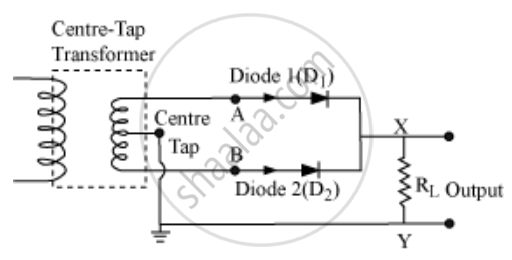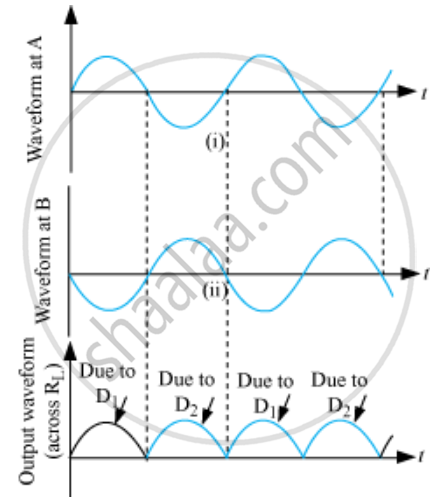Share

# A Student Wants to Use Two P-n Junction Diodes to Convert Alternating Current into Direct Current. Draw the Labelled Circuit Diagram She Would Use and Explain How It Works. - CBSE (Science) Class 12 - Physics

#### Question

A student wants to use two p-n junction diodes to convert alternating current into direct current. Draw the labelled circuit diagram she would use and explain how it works.

#### Solution

The student will use a full wave rectifier.

Two diodes are used to give rectified O/P corresponding to both positive as well as negative half cycles.When the voltage at A with respect to the centre tap is positive, and the voltage at B is negative. Then, D1 is forward biased and D2 is reversed biased. Hence, D1 conducts and D2 does not.

When the voltage of A becomes negative, then B becomes + ve. Therefore, D1 does not conduct and D2 conducts. Hence, we obtain output voltage during both the positive as well as negative half of cycle.Is there an error in this question or solution?
Solution A Student Wants to Use Two P-n Junction Diodes to Convert Alternating Current into Direct Current. Draw the Labelled Circuit Diagram She Would Use and Explain How It Works. Concept: p-n Junction.
S